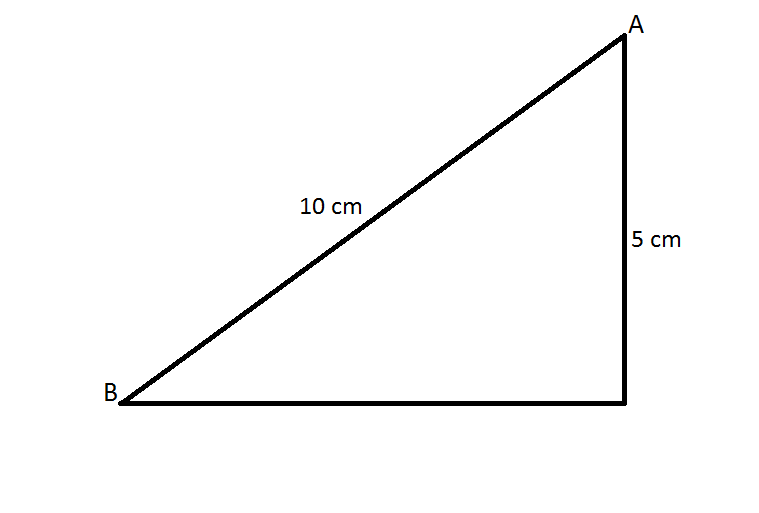# PhysicsThe above picture shows the image of an incline. Divide the incline $AB$ into $3$ parts such that if an object is released from the top (point $A$), it takes an equal amount of time $t$ to move through these parts. Then input your answer as $3t$

Sorry about the image, but the dimensions are $10m$ and $5m$

Take gravity to be $10m/{s}^{2}$

×